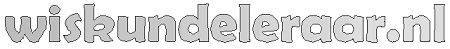Naschrift

Having found this relatively laborious solution, I stumbled across a truly remarkable formula for the area of an arbitrary quadrilateral. This is known as Brahmagupta's formula.

For a quadrilateral with sides a, b, c, d, semi-perimeter s, and for which q is half the sum of two opposite angles (it doesn't matter which pair), the area is given by:

A = Å$\sqrt{(s−a)(s−b)(s−c)(s−d)−abcd\cdot cos^{2}q}$

For a cyclic quadrilateral, i.e., a quadrilateral that can be inscribed in a circle, and for which the sum of opposite angles is $180^{o}$, $cos{q}=0$, thereby maximizing the area.

From this formula, the answer of $2\sqrt{6}$ drops straight out. Of course, a lot of work is embodied in that formula!